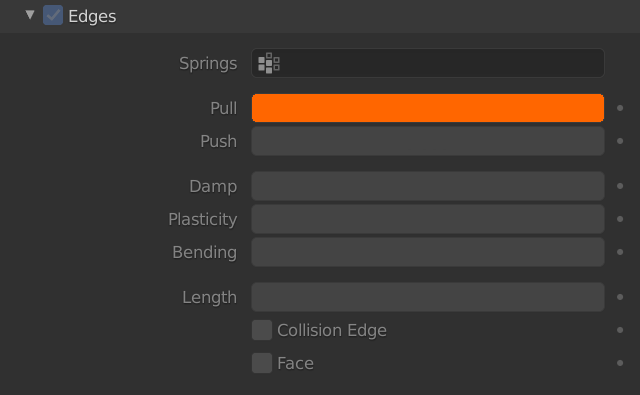# Pull

– This parameter let you set the spring stiffness for edges (how much the edges are allowed to stretch).
– In a Soft Body each edge is considered as a spring (no stretch and no squeeze in the original edge length which you designed as a part of your mesh):– A low value means very weak springs (a very elastic material).
– A high value is a strong spring (a stiffer material) that resists being pulled apart.

– In this example I created five vertices connected by one edge. See how different values of Pull affect the stiffness of five five edges (visualized here as springs).
– The vertices on the top are fixed (weight=1).
– The vertices on the bottom are free to move (weight=0) under the gravity force.